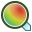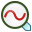# Impedance Goal

Optimise the impedance or admittance of a voltage or current source that is solved as part of the Feko model.

On the Request tab, in the Optimisation group, click theAdd goal function icon. From the drop down list, selectImpedance goal.

The Focus source label is identified based on the label of a voltage source or current source in CADFEKO or a card-defined source, for example, the A1, A2, A3, AF and AN card in EDITFEKO.

## Focus Types

The following focus types are available:

Both of these are complex quantities that represent the load characteristics (based on the currents and voltages at the source points). As these focus types always consist of complex values, the focus processing options require that there be at least one general processing step indicating the selection of one of the complex components.
Reflection coefficient (S11)
The reflection coefficient is computed with respect to the Reference impedance. For the impedance goal, the reflection coefficient is computed directly from the observed input impedance. This value is then in effect the “active” reflection coefficient ( $\Gamma$ ) and may differ from the S11 computed during an S-parameter calculation in a multi-port model.
Transmission coefficient
The transmission coefficient ( $1-\Gamma$ ) is considered with respect to the Reference impedance.
VSWR
The voltage standing wave ratio ( $\text{VSWR}=\frac{1+|{\text{S}}_{nn}|}{1-|{\text{S}}_{nn}|}$ ) for the observed input impedance is considered with respect to the Reference impedance.
Return losses
The return loss ( $-20\mathrm{log}|\Gamma |$ ) for the observed input impedance is considered with respect to the Reference impedance.
Current
The current flowing through the segment on which the selected voltage source is located. In order to use this optimisation goal, a port with a source or an A1 card must be applied to the segment of interest. The source should be set to zero magnitude, and a suitable Source name (label) entered.

## Reference Impedance

The impedance to be used during the calculation of the relevant focus types can be specified here. The impedance must be a single non-complex value and is local to each impedance goal (different reference impedances may be used for different impedance goals in the same optimisation search).